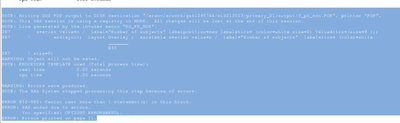## presenting n values outside xaxis using axistable statement

Im using the following code to populate the n values below each x axis ticks (outside the graph.). But I get the following error:How to handle this error and present the n values outside below the graph?

The code I'm using is below:

proc template;
define statgraph meanplot;
begingraph;
EntryTitle halign=left "Protocol: 213003 (HPTN 084-01)" halign=right "Page 1 of 1" /textattrs=(size=10pt weight=normal);
EntryTitle halign=left "Population: &g_poplbl" / textattrs=(size=10pt weight=normal);
EntryTitle "Figure &g_dsplynum" / textattrs=(size=10pt weight=normal );
EntryTitle "&g_title1" / textattrs=(size=10pt weight=normal);
EntryTitle "&g_title2" / textattrs=(size=10pt weight=normal);

legenditem type=line name="lgnd2" / lineattrs=(color=orange pattern=1 thickness=3) label="CAB (n=&nval)"; ** to adjust the size of the legend***;

layout overlay / xaxisopts=(labelFitPolicy=Split) xaxisopts=( Label="Weeks from Randomization" labelFitPolicy=Split TickValueAttrs=( Size=7) type=linear linearopts=( tickvaluelist=( 5 6 7 8 9 10 11 12 13 14 15 16 17 18 19 20 21 22 23 24 25 26 27 28
29 30 31 32 33 34 35 36 37 38 39 40 41 42 ) viewmin=5 viewmax=42 tickdisplaylist=( "Week 5" "Week 6" "" "" "Week 9" "Week 10" "" "" "" "" "" "" "Week 17" "Week 18" "" "" "" "" "" "" "Week 25" "Week 26" "" "" "" "" "" "" "Week 33" "Week 34" "" "" "" "" ""
"" "Step 3 Week +8" "") TickValueFitPolicy=RotateThin TickValueRotation=Diagonal ) )

yaxisopts=( Label="Plasma CAB (mcg/mL)" type=log logopts=( Base=10 TickIntervalStyle=LogExpand ) ) ;

seriesplot x=avisn y=aval_median/group=trt01ax NAME="lgnd" lineattrs=(color=orange pattern=1 thickness=1);
scatterPlot x=avisn y=aval_median/group=trt01a groupdisplay=cluster YErrorUpper=aval_p95 YErrorLower=aval_p5 NAME="lgnd1";

discretelegend "lgnd2" / title="" ;

ReferenceLine y=1.35 / clip=true Lineattrs=( Color=grey Pattern=1 Thickness=0.01) CURVELABEL="1.35 mcg/mL" CurveLabelAttrs=( Color=grey Size=7 ) CurveLabelLocation=Inside CurveLabelPosition=Max;

ReferenceLine y=0.65 / clip=true Lineattrs=( Color=grey Pattern=1 Thickness=0.01) CURVELABEL="0.65 mcg/mL" CurveLabelAttrs=( Color=grey Size=7 ) CurveLabelLocation=Inside CurveLabelPosition=Max;

ReferenceLine y=0.166 / clip=true Lineattrs=( Color=grey Pattern=1 Thickness=0.01) CURVELABEL="0.166 mcg/mL" CurveLabelAttrs=( Color=grey Size=7 ) CurveLabelLocation=Inside CurveLabelPosition=Max;

***axistable x=avisn value=n / Label="Number of subjects" labelposition=max labelattrs= (color=white size=0) ValueAttrs=(size=9 );;

endlayout;

Layout Overlay ;
axistable x=avisn value=n / Label="Number of subjects" labelattrs= (color=white size=0) ValueAttrs=(size=9 );
endlayout;

entryfootnote textattrs=(size=.0001pt color=white) "." ;
entryfootnote textattrs=(size=.0001pt color=white) "." ;
entryfootnote textattrs=(size=.0001pt color=white) "." ;
entryfootnote textattrs=(size=.0001pt color=white) "." ;
entryfootnote halign=left textattrs=(size=10pt ) "&g_foot1" ;
entryfootnote halign=left textattrs=(size=10pt ) "&g_foot2" ;
entryfootnote halign=left textattrs=(size=10pt ) "&g_foot3" ;
entryfootnote halign=left textattrs=(size=10pt ) "&g_foot4" ;
entryfootnote halign=left textattrs=(size=10pt ) "&g_foot5" ;
entryfootnote halign=left textattrs=(size=10pt ) "&g_foot6" ;
entryfootnote halign=left textattrs=(size=10pt ) "&g_foot7" ;
entryfootnote halign=left textattrs=(size=10pt ) "&g_foot8" ;
entryfootnote halign=left textattrs=(size=10pt ) "&g_foot9" ;
entryfootnote halign=left textattrs=(size=10pt ) "&g_userid: &g_pgmpth &sysdate9 &systime" ;

endgraph;
end;
run;

1 ACCEPTED SOLUTION

Accepted SolutionsDanH_sas
SAS Super FREQ

## Re: presenting n values outside xaxis using axistable statement

The problem is that you have two root layouts -- both of them LayoutOverlay's. I think you intent was to put the axis table outside of the data area. To do that, you need to wrap both LayoutOverlay's with a LayoutLattice. Something like the following:

``````begingraph;
layout lattice / columnweights=preferred rowweights=preferred columndatarange=union columns=1 columnweights=preferred;
layout overlay;
endlayout;
Layout Overlay /  walldisplay=none xaxisopts=(display=none);
AxisTable VALUE=<tablevar1> X=<xvar> / labelPosition=min Display=(Label);
endlayout;
Layout Overlay /  walldisplay=none xaxisopts=(display=none);
AxisTable VALUE=<tablevar2> X=<xvar> / labelPosition=min Display=(Label);
endlayout;
endlayout;
endgraph;``````

Hope this helps!

Dan

3 REPLIES 3

## Re: presenting n values outside xaxis using axistable statement

When you get an error include ALL of the text from the data step or Proc statement that generates the error. Copy the text from the log and paste into a text box opened on the forum with the </> icon that appears above the message window.

Similar for code, paste into a text box. The message windows will reformat text and sometimes make changes that result in code that will not run in addition to being ugly and hard to read.

I can't even read your picture because there is not enough contrast between the text and background color.DanH_sas
SAS Super FREQ

## Re: presenting n values outside xaxis using axistable statement

The problem is that you have two root layouts -- both of them LayoutOverlay's. I think you intent was to put the axis table outside of the data area. To do that, you need to wrap both LayoutOverlay's with a LayoutLattice. Something like the following:

``````begingraph;
layout lattice / columnweights=preferred rowweights=preferred columndatarange=union columns=1 columnweights=preferred;
layout overlay;
endlayout;
Layout Overlay /  walldisplay=none xaxisopts=(display=none);
AxisTable VALUE=<tablevar1> X=<xvar> / labelPosition=min Display=(Label);
endlayout;
Layout Overlay /  walldisplay=none xaxisopts=(display=none);
AxisTable VALUE=<tablevar2> X=<xvar> / labelPosition=min Display=(Label);
endlayout;
endlayout;
endgraph;``````

Hope this helps!

Dan

## Re: presenting n values outside xaxis using axistable statement

Hi @DanH_sas
Thank you for the solution, You guessed right and your method works.

Discussion stats
• 3 replies
• 305 views
• 0 likes
• 3 in conversation